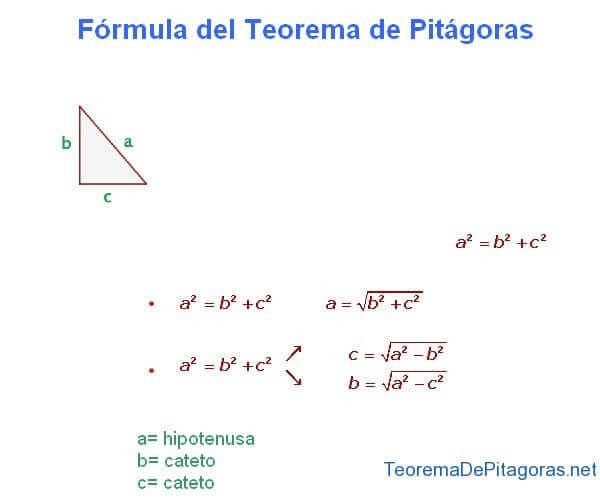# Formula of the Pythagorean Theorem

TOPICS: Right Angle     Leg     Legs     Pythagoras Formula     Hypotenuse     Right Triangle

Next we’re going to look at the formula of the Pythagorean Theorem, because of all the knowledge that Pythagoras left us regarding the proportions of the sides of a right triangle, without a doubt the most important is the formula of his theorem itself, a formula that we have all had to learn at some point in our lives and which, beyond that, is actually very useful for many situations where we can find a practical application for it.

The Pythagorean Theorem says that, in a right triangle, the hypotenuse is equal to the square root of the sum of the squares of the legs and that, in the same type of triangle, one of the legs is equal to the square root of the hypotenuse squared minus the other leg squared. But today we’re not going to worry about theory, but instead to visually see where the formula of the Pythagorean Theorem comes from.

In the image below, you can see the formula of the Pythagorean Theorem, also graphically showing each one of the sides of a right triangle, the hypotenuse (the longest side which is opposite the right angle) and the two legs (the sides that form the right angle). In this case we have called the hypotenuse “a” and the two legs “b” and “c”.